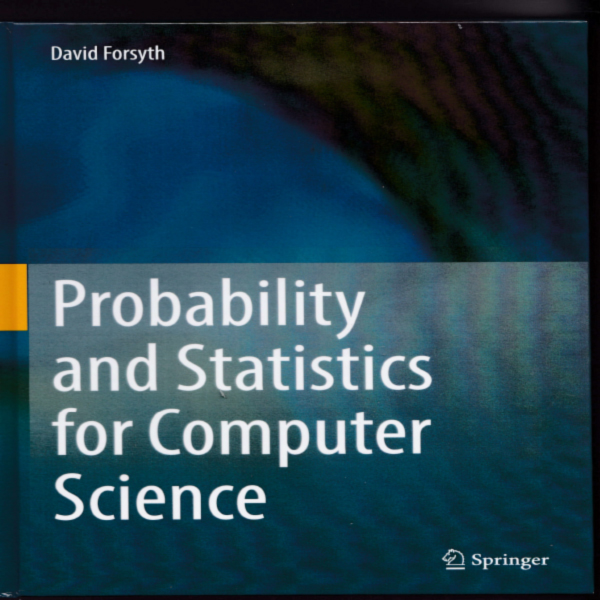# Probability and Statistics for Computer Scientists## by David Forsyth

daf@uiuc.edu, daf@illinois.edu

## What's in it

I taught a course several times at UIUC to undergrad computer science students. The remit of the course was to teach the bits of probability and statistics that all computer science students should know. As Molesworth used to say, "Akwire kulchur and kepe the brane kleen" (spelling from memory; thanks Geoffrey Willans and Ronald Searle). I taught some simple data visualization methods; some discrete probability; some continuous probability; principal components analysis; some inference; some classification; some clustering; and some regression. The book is a cleaned up version of my notes from that course. I placed a lot of weight on using real data sets, and on getting a broad coverage.

• Describing Data
• First tools for looking at data
• Looking at relationships
• Probability
• Basic ideas in probability
• Random variables and expectations
• Useful probability distributions
• Inference
• Samples and populations
• The significance of evidence
• Experiments
• Inferring probability models from data
• Tools
• Extracting important relationships in high dimensions
• Learning to classify
• Clustering: models of high dimensional data
• Regression
• Markov chains and hidden markov models